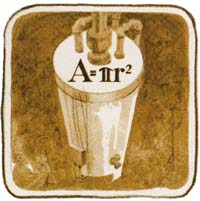Section 7: Simple Programming110
 One value you needed in order to calculate the heat loss from the water heater was the surface area of the cylinder. Let’s begin the next problem by calculating just the surface area of the top of the cylinder, which, of course, is a circle. The formula for the area of a circle is A = πr2.To calculate the area of the circle manually you would first key in the radius r, and then square it by pressing g X2 . Next you would press g   to summon the quantity pi. Finally you would multiply the squared radius and the quantity pi together by pressing  ×  .
Remember that a program is little more than the keystrokes you would press to solve the problem manually. So, in the program, the keys you press to solve the problem are the same as the keys you press to solve the problem manually. You will load these keystrokes into program memory:
 g X2 g ×
In addition, your program will contain two other operations, LBL and END .
The Beginning of a Program
The beginning of each program you write should be ‘‘named’’ or labeled with a string of ALPHA characters, or a two-digit number. These program labels enable you to keep track of, and easily use, the programs you write. In a few moments you will learn how to use LBL label to label your programs. First, there are a few things that you should know about labels.
Program labels that are ALPHA characters can consist of any seven ALPHA characters except , (comma), . (period), : (colon).
Used as program labels, the single letters A through J and a through e have a special ‘‘local label’’ function in the HP-41C. These letters should not be used as the first label in your program. They are most useful when used inside programs. Don’t be concerned with local labels now—they are covered in detail in section 12. For now, just remember not to label your main programs with A through J and a through e.
Program labels that are numbers must be two digits. Number labels are most often used to label subroutines. Use of numeric labels is covered later.